Home Practice
For learners and parents For teachers and schools
Textbooks
Full catalogue
Pricing SupportLog in

We think you are located in United States. Is this correct?

# 6.4 Hyperbolic functions

## 6.4 Hyperbolic functions (EMA4P)

### Functions of the form $$y=\dfrac{1}{x}$$ (EMA4Q)

Functions of the general form $$y=\dfrac{a}{x}+q$$ are called hyperbolic functions.

## Worked example 8: Plotting a hyperbolic function

$$y=h\left(x\right)=\dfrac{1}{x}$$

Complete the following table for $$h\left(x\right)=\dfrac{1}{x}$$ and plot the points on a system of axes.

 $$x$$ $$-\text{3}$$ $$-\text{2}$$ $$-\text{1}$$ $$-\dfrac{1}{2}$$ $$-\dfrac{1}{4}$$ 0 $$\dfrac{1}{4}$$ $$\dfrac{1}{2}$$ $$\text{1}$$ $$\text{2}$$ $$\text{3}$$ $$h\left(x\right)$$ $$-\dfrac{1}{3}$$
1. Join the points with smooth curves.

2. What happens if $$x = 0$$?

3. Explain why the graph consists of two separate curves.

4. What happens to $$h\left(x\right)$$ as the value of $$x$$ becomes very small or very large?

5. The domain of $$h\left(x\right)$$ is $$\left\{x:x\in \mathbb{R},x\ne 0\right\}$$. Determine the range.

6. About which two lines is the graph symmetrical?

### Substitute values into the equation

\begin{align*} h(x)& = \frac{1}{x} \\ h(-3)& = \frac{1}{-3} = -\frac{1}{3} \\ h(-2)& = \frac{1}{-2} = -\frac{1}{2} \\ h(-1)& = \frac{1}{-1} = -1 \\ h\left(-\frac{1}{2}\right)& = \frac{1}{-\frac{1}{2}} = -2 \\ h\left(-\frac{1}{4}\right)& = \frac{1}{-\frac{1}{4}} = -4 \\ h(0)& = \frac{1}{0} = \text{undefined} \\ h\left(\frac{1}{4}\right)& = \frac{1}{\frac{1}{4}} = 4 \\ h\left(\frac{1}{2}\right)& = \frac{1}{\frac{1}{2}} = 2 \\ h(1)& = \frac{1}{1} = 1 \\ h(2)& = \frac{1}{2} = \frac{1}{2} \\ h(3)& = \frac{1}{3} = \frac{1}{3} \end{align*}
 $$x$$ $$-\text{3}$$ $$-\text{2}$$ $$-\text{1}$$ $$-\dfrac{1}{2}$$ $$-\dfrac{1}{4}$$ $$\text{0}$$ $$\dfrac{1}{4}$$ $$\dfrac{1}{2}$$ $$\text{1}$$ $$\text{2}$$ $$\text{3}$$ $$h(x)$$ $$-\dfrac{1}{3}$$ $$-\dfrac{1}{2}$$ $$-\text{1}$$ $$-\text{2}$$ $$-\text{4}$$ undefined $$\text{4}$$ $$\text{2}$$ $$\text{1}$$ $$\dfrac{1}{2}$$ $$\dfrac{1}{3}$$

### Plot the points and join with two smooth curves

From the table we get the following points: $$\left(-3;-\frac{1}{3}\right)$$, $$\left(-2;-\frac{1}{2}\right)$$, $$\left(-1;-1\right)$$, $$\left(-\frac{1}{2};-2\right)$$, $$\left(-\frac{1}{4};-4\right)$$, $$\left(\frac{1}{4};4\right)$$, $$\left(\frac{1}{2};2\right)$$, $$\left(1;1\right)$$, $$\left(2;\frac{1}{2}\right)$$, $$\left(3;\frac{1}{3}\right)$$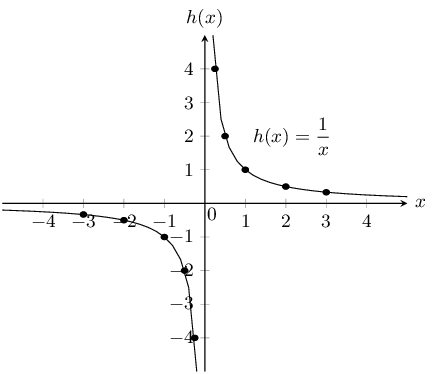For $$x=0$$ the function $$h$$ is undefined. This is called a discontinuity at $$x=0$$.

$$y=h(x)=\dfrac{1}{x}$$ therefore we can write that $$x\times y=1$$. Since the product of two positive numbers and the product of two negative numbers can be equal to $$\text{1}$$, the graph lies in the first and third quadrants.

### Determine the asymptotes

As the value of $$x$$ gets larger, the value of $$h(x)$$ gets closer to, but does not equal $$\text{0}$$. This is a horizontal asymptote, the line $$y=0$$. The same happens in the third quadrant; as $$x$$ gets smaller $$h(x)$$ also approaches the negative $$x$$-axis asymptotically.

We also notice that there is a vertical asymptote, the line $$x=0$$; as $$x$$ gets closer to $$\text{0}$$, $$h(x)$$ approaches the $$y$$-axis asymptotically.

### Determine the range

Domain: $$\left\{x:x\in \mathbb{R},x\ne 0\right\}$$

From the graph, we see that $$y$$ is defined for all values except $$\text{0}$$.

Range: $$\left\{y:y\in \mathbb{R},y\ne 0\right\}$$

### Determine the lines of symmetry

The graph of $$h(x)$$ has two axes of symmetry: the lines $$y=x$$ and $$y=-x$$. About these two lines, one half of the hyperbola is a mirror image of the other half.

## The effects of $$a$$ and $$q$$ on a hyperbola.

On the same set of axes, plot the following graphs:

1. $${y}_{1}=\dfrac{1}{x}-2$$

2. $${y}_{2}=\dfrac{1}{x}-1$$

3. $${y}_{3}=\dfrac{1}{x}$$

4. $${y}_{4}=\dfrac{1}{x}+1$$

5. $${y}_{5}=\dfrac{1}{x}+2$$

Use your results to deduce the effect of $$q$$.

On the same set of axes, plot the following graphs:

1. $${y}_{6}=\dfrac{-2}{x}$$

2. $${y}_{7}=\dfrac{-1}{x}$$

3. $${y}_{8}=\dfrac{1}{x}$$

4. $${y}_{9}=\dfrac{2}{x}$$

Use your results to deduce the effect of $$a$$.

The effect of $$q$$

The effect of $$q$$ is called a vertical shift because all points are moved the same distance in the same direction (it slides the entire graph up or down).

• For $$q>0$$, the graph of $$f(x)$$ is shifted vertically upwards by $$q$$ units.

• For $$q<0$$, the graph of $$f(x)$$ is shifted vertically downwards by $$q$$ units.

The horizontal asymptote is the line $$y = q$$ and the vertical asymptote is always the $$y$$-axis, the line $$x = 0$$.

The effect of $$a$$

The sign of $$a$$ determines the shape of the graph.

• If $$a > 0$$, the graph of $$f(x)$$ lies in the first and third quadrants.

For $$a > 1$$, the graph of $$f(x)$$ will be further away from the axes than $$y = \dfrac{1}{x}$$.

For $$0 < a < 1$$, as $$a$$ tends to $$\text{0}$$, the graph moves closer to the axes than $$y = \dfrac{1}{x}$$.

• If $$a < 0$$, the graph of $$f(x)$$ lies in the second and fourth quadrants.

For $$a < -1$$, the graph of $$f(x)$$ will be further away from the axes than $$y = -\dfrac{1}{x}$$.

For $$-1 < a < 0$$, as $$a$$ tends to $$\text{0}$$, the graph moves closer to the axes than $$y=-\dfrac{1}{x}$$.

 $$a<0$$ $$a>0$$ $$q>0$$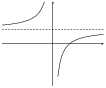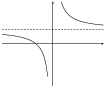$$q=0$$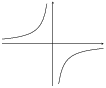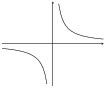$$q<0$$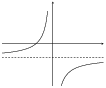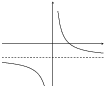Table 6.3: The effects of $$a$$ and $$q$$ on a hyperbola.

### Discovering the characteristics (EMA4S)

The standard form of a hyperbola is the equation $$y=\dfrac{a}{x}+q$$.

#### Domain and range

For $$y = \dfrac{a}{x} + q$$, the function is undefined for $$x=0$$. The domain is therefore $$\left\{x:x\in \mathbb{R}, x\ne 0\right\}$$.

We see that $$y = \dfrac{a}{x} + q$$ can be rewritten as:

\begin{align*} y& = \frac{a}{x}+q \\ y-q& = \frac{a}{x} \\ \text{If } x\ne 0 \text{ then: }\left(y-q\right)x& = a \\ x& = \frac{a}{y-q} \end{align*}

This shows that the function is undefined only at $$y = q$$.

Therefore the range is $$\left\{f(x):f(x)\in \mathbb{R}, f(x)\ne q\right\}$$

## Worked example 9: Domain and range of a hyperbola

If $$g(x) = \dfrac{2}{x}+2$$, determine the domain and range of the function.

### Determine the domain

The domain is $$\left\{x:x\in \mathbb{R}, x\ne 0\right\}$$ because $$g(x)$$ is undefined only at $$x=0$$.

### Determine the range

We see that $$g(x)$$ is undefined only at $$y = 2$$. Therefore the range is $$\left\{g(x):g(x)\in \mathbb{R}, g(x)\ne 2\right\}$$

#### Intercepts

The $$y$$-intercept:

Every point on the $$y$$-axis has an $$x$$-coordinate of $$\text{0}$$, therefore to calculate the $$y$$-intercept let $$x = 0$$.

For example, the $$y$$-intercept of $$g(x) = \dfrac{2}{x}+2$$ is given by setting $$x = 0$$:

\begin{align*} y& = \frac{2}{x}+2 \\ y& = \frac{2}{0}+2 \end{align*}

which is undefined, therefore there is no $$y$$-intercept.

The $$x$$-intercept:

Every point on the $$x$$-axis has a $$y$$-coordinate of $$\text{0}$$, therefore to calculate the $$x$$-intercept, let $$y = 0$$.

For example, the $$x$$-intercept of $$g(x)=\dfrac{2}{x}+2$$ is given by setting $$y = 0$$:

\begin{align*} y& = \frac{2}{x}+2 \\ 0& = \frac{2}{x}+2 \\ \frac{2}{x}& = -2 \\ x& = \frac{2}{-2} \\ & = -1 \end{align*}

This gives the point $$(-1;0)$$.

#### Asymptotes

There are two asymptotes for functions of the form $$y = \dfrac{a}{x} + q$$.

The horizontal asymptote is the line $$y = q$$ and the vertical asymptote is always the $$y$$-axis, the line $$x = 0$$.

#### Axes of symmetry

There are two lines about which a hyperbola is symmetrical: $$y = x + q$$ and $$y = -x + q$$.

### Sketching graphs of the form $$y = \dfrac{a}{x} + q$$ (EMA4T)

In order to sketch graphs of functions of the form, $$y=f(x) = \dfrac{a}{x} + q$$, we need to determine four characteristics:

1. sign of $$a$$

2. $$y$$-intercept

3. $$x$$-intercept

4. asymptotes

## Worked example 10: Sketching a hyperbola

Sketch the graph of $$g(x) = \dfrac{2}{x} + 2$$. Mark the intercepts and the asymptotes.

### Examine the standard form of the equation

We notice that $$a > 0$$ therefore the graph of $$g(x)$$ lies in the first and third quadrant.

### Calculate the intercepts

For the $$y$$-intercept, let $$x = 0$$:

\begin{align*} g(x)=& \frac{2}{x}+2 \\ g(0)=& \frac{2}{0}+2 \end{align*}

This is undefined, therefore there is no $$y$$-intercept.

For the $$x$$-intercept, let $$y = 0$$:

\begin{align*} g(x)& = \frac{2}{x}+2 \\ 0& = \frac{2}{x}+2 \\ \frac{2}{x}& = -2 \\ \therefore x& = -1 \end{align*}

This gives the point $$(-1;0)$$.

### Determine the asymptotes

The horizontal asymptote is the line $$y = 2$$. The vertical asymptote is the line $$x = 0$$.

### Sketch the graph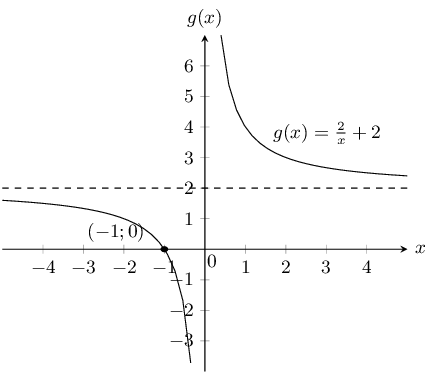Domain: $$\left\{x:x\in \mathbb{R}, x\ne 0\right\}$$

Range: $$\left\{y:y\in \mathbb{R}, y\ne 2\right\}$$

## Worked example 11: Sketching a hyperbola

Sketch the graph of $$y = \dfrac{-4}{x} + 7$$.

### Examine the standard form of the equation

We see that $$a < 0$$ therefore the graph lies in the second and fourth quadrants.

### Calculate the intercepts

For the $$y$$-intercept, let $$x = 0$$:

\begin{align*} y& = \frac{-4}{x}+7 \\ & = \frac{-4}{0}+7 \end{align*}

This is undefined, therefore there is no $$y$$-intercept.

For the $$x$$-intercept, let $$y = 0$$:

\begin{align*} y& = \frac{-4}{x}+7 \\ 0& = \frac{-4}{x}+7 \\ \frac{-4}{x}& = -7 \\ \therefore x& = \frac{4}{7} \end{align*}

This gives the point $$\left(\dfrac{4}{7};0\right)$$.

### Determine the asymptotes

The horizontal asymptote is the line $$y = 7$$. The vertical asymptote is the line $$x = 0$$.

### Sketch the graph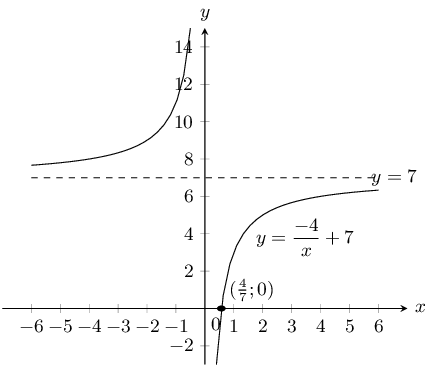Domain: $$\left\{x:x\in \mathbb{R}, x\ne 0\right\}$$

Range: $$\left\{y:y\in \mathbb{R}, y\ne 7\right\}$$

Axis of symmetry: $$y=x+7$$ and $$y=-x+7$$

Textbook Exercise 6.4

The following graph shows a hyperbolic equation of the form $$y = \dfrac {a}{x} + q$$. Point A is shown at $$\left( -2 ; \dfrac{5}{2} \right)$$. Calculate the values of $$a$$ and $$q$$.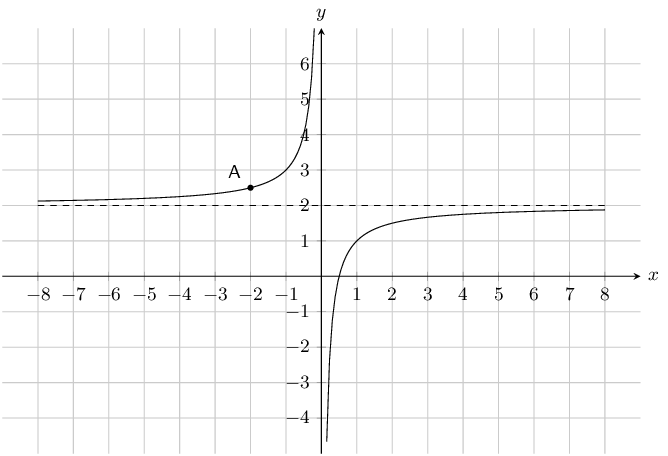\begin{align*} q & = 2 \\ \\ y & = \frac {a}{x} +2 \\ \left( \frac{5}{2} \right) & = \dfrac {a}{(-2)} +2 \\ -2 \left( \frac{5}{2} \right) & = \left[ \dfrac {a}{-2} +2 \right] (-2) \\ -\text{5} & = a -\text{4} \\ -\text{1} & = a \end{align*}

Therefore $a = -1$ and $$q = 2$$

The equation is $$y = - \dfrac {1}{x} +2$$.

The following graph shows a hyperbolic equation of the form $$y = \dfrac {a}{x} + q$$. Point A is shown at $$\left( -1 ; 5 \right)$$. Calculate the values of $$a$$ and $$q$$.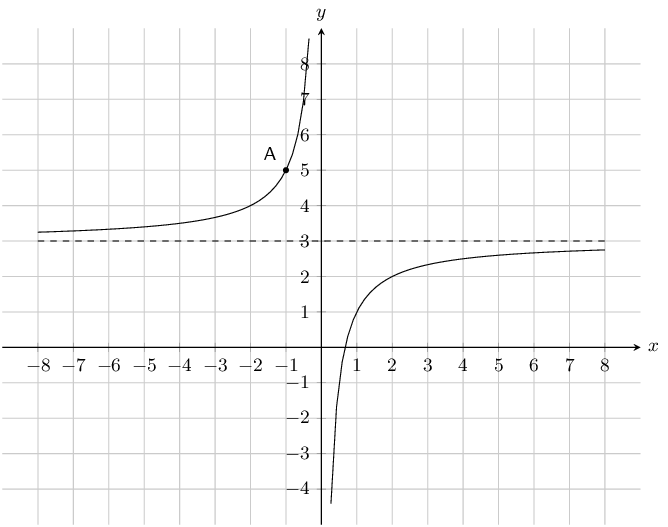\begin{align*} q & = 3 \\ \\ y & = \frac {a}{x} +3 \\ (5) & = \dfrac {a}{(-1)} +3 \\ -1(5) & = \left[ \dfrac {a}{-1} +3 \right](-1) \\ -\text{5} & = a-\text{3} \\ -\text{2} & = a \end{align*}

Therefore $a = -2$ and $$q = 3$$

The equation is $$y = - \dfrac {2}{x} +3$$.

Given the following equation: $y = \frac{3}{x} +2$

Determine the location of the $$y$$-intercept.

\begin{align*} y & = \frac{3}{x} +2 \\ & = \frac{3}{(0)} +2\\ & \text{undefined} \end{align*}

There is no $$y$$-intercept.

Determine the location of the $$x$$-intercept. Give your answer as a fraction.

\begin{align*} y & = \frac{3}{x} +2 \\ (0) & = \frac{3}{x} +2 \\ (x)(0) & = \left[ \frac{3}{x} +2 \right](x) \\ 0 & = 3 +2x \\ -3 & = 2 x \\ x & = - \frac{3}{2} \end{align*}

Given the following equation: $y = - \frac{2}{x} -2$

Determine the location of the $$y$$-intercept.

\begin{align*} y & = - \frac{2}{x} -2 \\ y & = - \frac{2}{(0)} -2\\ & \text{undefined} \end{align*}

There is no $$y$$-intercept.

Determine the location of the $$x$$-intercept.

\begin{align*} y & = - \frac{2}{x} -2 \\ (0) & = - \frac{2}{x} -2 \\ (x)(0) & = \left[-\frac{2}{x} -2 \right](x) \\ 0 & = -2 - 2x \\ 2 & = -2x \\ x & = -1 \end{align*}

Given the following graph, identify a function that matches each of the following equations: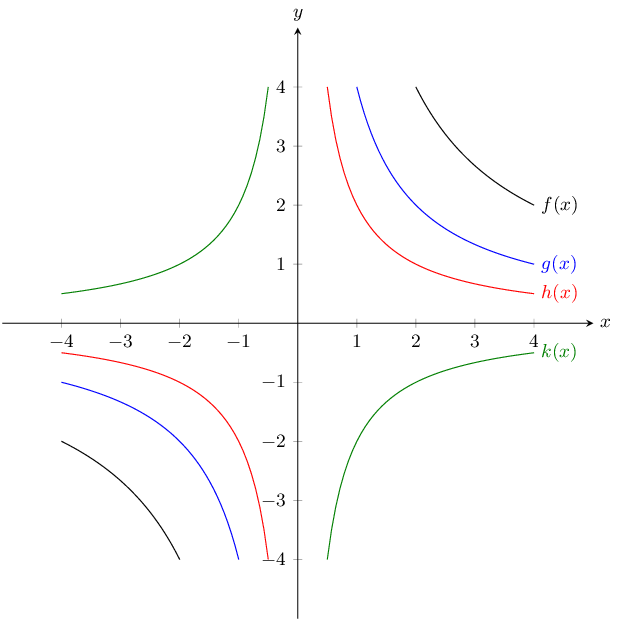$$y = \dfrac{2}{x}$$

$$h(x)$$

$$y = \dfrac{4}{x}$$

$$g(x)$$

$$y = -\dfrac{2}{x}$$

$$k(x)$$

$$y = \dfrac{8}{x}$$

$$f(x)$$

Given the function: $$xy=-6$$.

Draw the graph.

$$a$$ is negative and so the function lies in the second and fourth quadrants.

There is no $$y$$-intercept or $$x$$-intercept.

Instead we can plot the graph from a table of values.

$\begin{array}{|c|c|c|c|c|} \hline x & -2 & -1 & 1 & 2 \\ \hline y & 3 & 6 & -6 & -3 \\ \hline \end{array}$

Now we can plot the graph: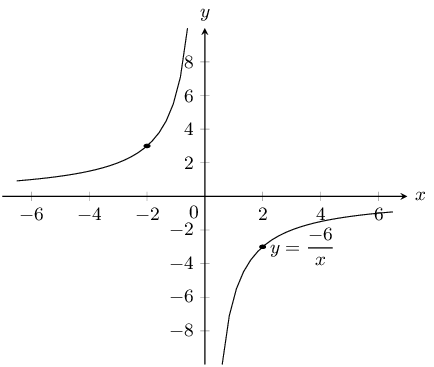Does the point $$(-2;3)$$ lie on the graph? Give a reason for your answer.

If we substitute the point $$(-2; 3)$$ into each side of the equation we get:

\begin{align*} RHS: & = -6 \\ LHS: & xy = (-2)(3) = -6 \end{align*}

This satisfies the equation therefore the point does lie on the graph.

If the $$x$$-value of a point on the graph is $$\text{0,25}$$ what is the corresponding $$y$$-value?

Substitute in the value of $$x$$: \begin{align*} y&=\frac{-6}{\text{0,25}} \\ & = \frac{-6}{\frac{1}{4}} \\ &= -6 \times \frac{4}{1} \\ &= -24 \end{align*}

What happens to the $$y$$-values as the $$x$$-values become very large?

The $$y$$-values decrease as the $$x$$-values become very large. The larger the denominator ($$x$$), the smaller the result of the fraction ($$y$$).

Give the equation of the asymptotes.

The graph is not vertically or horizontally shifted, therefore the asymptotes are $$y=0$$ and $$x=0$$.

With the line $$y=-x$$ as a line of symmetry, what is the point symmetrical to $$(-2;3)$$?

Across the line of symmetry $$y=-x$$, the point symmetrical to $$(-2; 3)$$ is $$(-3;2)$$.

Given the function: $$h(x)=\dfrac{8}{x}$$.

Draw the graph.

$$a$$ is positive and so the function lies in the first and third quadrants.

There is no $$y$$-intercept and no $$x$$-intercept.

Instead we can plot the graph from a table of values.

$\begin{array}{|c|c|c|c|c|} \hline x & -2 & -1 & 1 & 2 \\ \hline y & -4 & -8 & 8 & 4 \\ \hline \end{array}$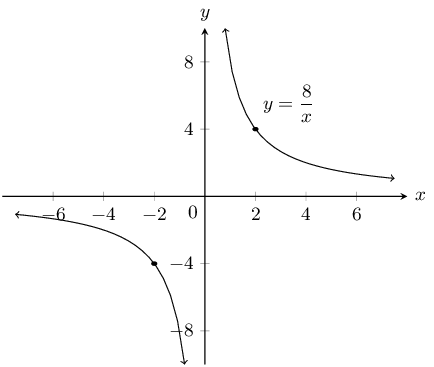How would the graph of $$g(x)=\dfrac{8}{x}+3$$ compare with that of $$h(x)=\dfrac{8}{x}$$? Explain your answer fully.

The graph $$g(x)=\dfrac{8}{x}+3$$ is the graph of $$h(x)=\dfrac{8}{x}$$, vertically shifted upwards by $$3$$ units. They would be the same shape but the asymptote of $$g(x)$$ would be $$y=3$$, instead of $$y=0$$ (for $$h(x)$$) and the axis of symmetry would be $$y=-x+3$$ instead of $$y = -x$$.

Draw the graph of $$y = \dfrac{8}{x} + 3$$ on the same set of axes, showing asymptotes, axes of symmetry and the coordinates of one point on the graph.

$$a$$ is positive and so the function lies in the first and third quadrants.

For $$y = \frac{8}{x} + 3$$ there is no $$y$$-intercept. The $$x$$-intercept is at $$-\frac{8}{3}$$.

We can plot the graph from a table of values.

$\begin{array}{|c|c|c|c|c|} \hline x & -4 & -2 & 2 & 4 \\ \hline y & 1 & -1 & 7 & 5 \\ \hline \end{array}$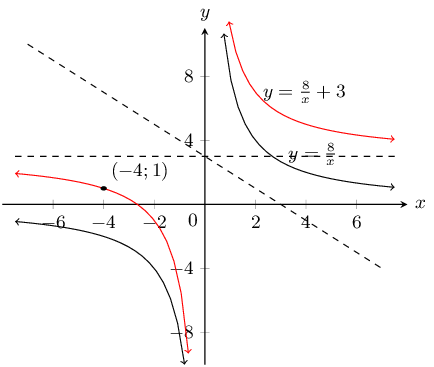Sketch the functions given and describe the transformation performed on the first function to obtain the second function. Show all asymptotes.

$$y = \dfrac{1}{x}$$ and $$\dfrac{3}{x}$$

$$a$$ is positive for both graphs and so both graphs lie in the first and third quadrants.

For both graphs there is no $$y$$-intercept or $$x$$-intercept.

Instead we can plot the graph from a table of values.

$$y = \frac{1}{x}$$:

$\begin{array}{|c|c|c|c|c|} \hline x & -2 & -1 & 1 & 2 \\ \hline y & -\frac{1}{2} & -1 & 1 & \frac{1}{2} \\ \hline \end{array}$

$$y = \frac{3}{x}$$:

$\begin{array}{|c|c|c|c|c|} \hline x & -2 & -1 & 1 & 2 \\ \hline y & -\frac{3}{2} & -3 & 3 & \frac{3}{2} \\ \hline \end{array}$

The asymptotes are $$y = 0$$ and $$x = 0$$.

Now we can plot the graphs: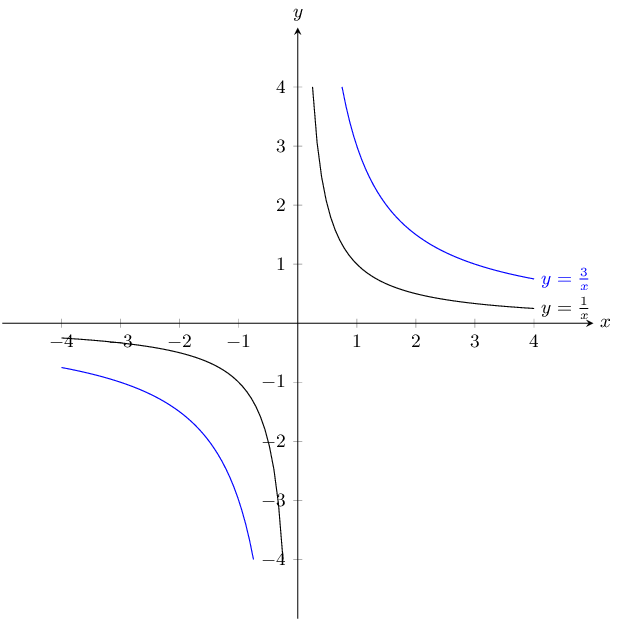Magnification by 3

$$y = \dfrac{6}{x}$$ and $$\dfrac{6}{x} - 1$$

$$a$$ is positive for both graphs and so both graphs lie in the first and third quadrants.

For both graphs there is no $$y$$-intercept. For $$y = \frac{6}{x}$$ there is no $$x$$-intercept. For $$y = \frac{6}{x} - 1$$ the $$x$$-intercept is $$(6;0)$$.

We can plot the graphs from a table of values.

$$y = \frac{6}{x}$$:

$\begin{array}{|c|c|c|c|c|} \hline x & -2 & -1 & 1 & 2 \\ \hline y & -3 & -6 & 6 & 3 \\ \hline \end{array}$

$$y = \frac{6}{x} - 1$$:

$\begin{array}{|c|c|c|c|c|} \hline x & -2 & -1 & 1 & 2 \\ \hline y & -4 & -7 & 5 & 2 \\ \hline \end{array}$

The asymptotes for $$y = \frac{6}{x}$$ are $$y = 0$$ and $$x = 0$$.

The asymptotes for $$y = \frac{6}{x} - 1$$ are $$y = -1$$ and $$x = 0$$.

Now we can plot the graphs: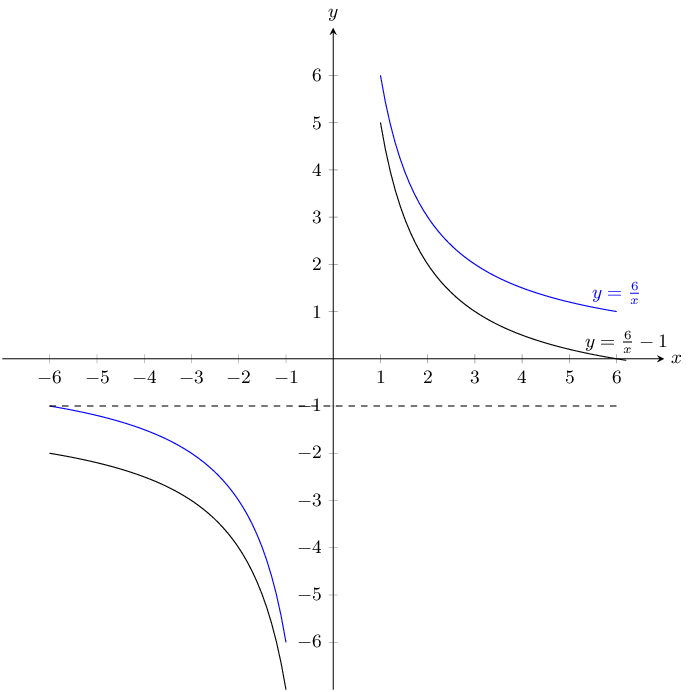Translation along the $$y$$-axis by -1

$$y = \dfrac{5}{x}$$ and $$-\dfrac{5}{x}$$

$$y = \frac{5}{x}$$:

$$a$$ is positive and so the graph lies in the first and third quadrants.

There is no $$y$$-intercept and no $$x$$-intercept.

We can plot the graph from a table of values.

$\begin{array}{|c|c|c|c|c|} \hline x & -2 & -1 & 1 & 2 \\ \hline y & -\frac{5}{2} & -5 & 5 & \frac{5}{2} \\ \hline \end{array}$

The asymptotes are $$y = 0$$ and $$x = 0$$.

$$y = -\frac{5}{x}$$:

$$a$$ is negative and so the graph lies in the second and fourth quadrants.

There is no $$y$$-intercept and no $$x$$-intercept.

We can plot the graph from a table of values.

$\begin{array}{|c|c|c|c|c|} \hline x & -2 & -1 & 1 & 2 \\ \hline y & \frac{5}{2} & 5 & -5 & -\frac{5}{2} \\ \hline \end{array}$

The asymptotes are $$y = 0$$ and $$x = 0$$.

Now we can plot the graphs: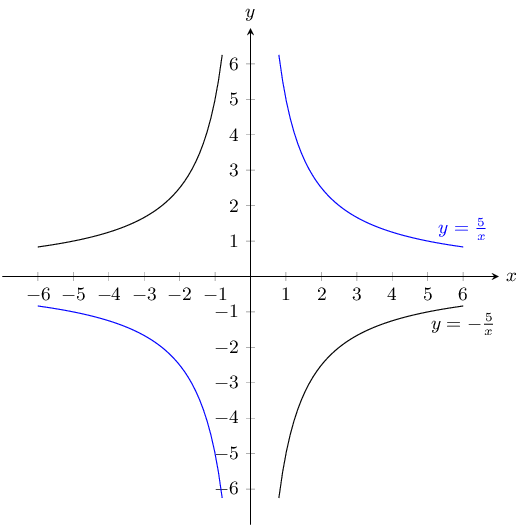Reflection on the $$x$$-axis or reflection on the $$y$$-axis.

$$y = \dfrac{1}{x}$$ and $$\dfrac{1}{2x}$$

$$a$$ is positive for both graphs and so both graphs lie in the first and third quadrants.

For both graphs there is no $$y$$-intercept and no $$x$$-intercept.

We can plot the graphs from a table of values.

$$y = \frac{1}{x}$$:

$\begin{array}{|c|c|c|c|c|} \hline x & -2 & -1 & 1 & 2 \\ \hline y & -\frac{1}{2} & -1 & 1 & \frac{1}{2} \\ \hline \end{array}$

$$y = \frac{1}{2x}$$:

$\begin{array}{|c|c|c|c|c|} \hline x & -2 & -1 & 1 & 2 \\ \hline y & -\frac{1}{4} & -\frac{1}{2} & \frac{1}{2} & \frac{1}{4} \\ \hline \end{array}$

The asymptotes for both graphs are $$y = 0$$ and $$x = 0$$.

Now we can plot the graphs: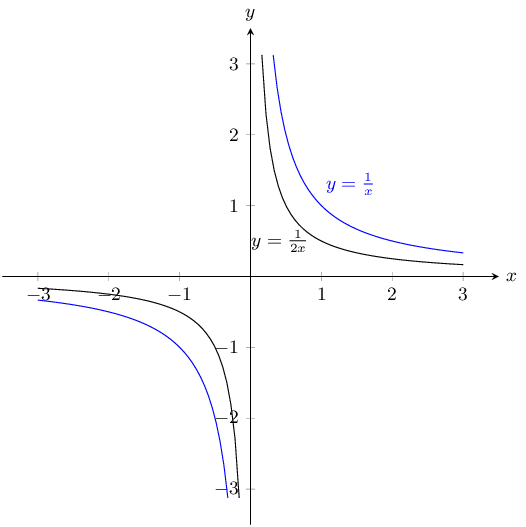Reduction by 2